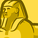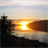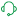Announcements
You can succeed best and quickest by helping others to succeed. Join the conversation.
cancel
Showing results for
Did you mean:Creator III

## Calculate % for respective month instead of overall

I need to calculate Percent of Volume By Month per category instead of percent overall.

Right now, my expression is as below:

Count(DISTINCT{<DATE_DT={"\$(='>=' & Date(AddMonths(Max(DATE_DT), -12)) & '<=' & Date(Max(DATE_DT))))"}>} ID)
/
Count(TOTAL DISTINCT ID)

The above expression calculates Percent of IDs for rolling 12 months and when I add Month Year Dimension to the chart, it shows Percent for overall volume.

I need to show Percentage for that respective month instead of overall time period.

Labels (3)

• ### Visualization

1 Solution

Accepted SolutionsSupport

Hello, @qlikwiz123

To calculate the percent of volume by month per category, you can modify the existing expression to include the Month Year dimension in the numerator and denominator of the expression. Here's an example expression that you can try to use and see if it will work:

Count(DISTINCT{<DATE_DT={"\$(='>=' & Date(AddMonths(Max(DATE_DT), -12)) & '<=' & Date(Max(DATE_DT))))"}>} ID * MonthYear) / Count(TOTAL DISTINCT {<MonthYear>} ID)

In this expression, you are multiplying the count of distinct IDs for each month by the Month Year dimension, which will give you the volume for each category by month. You are then dividing this by the total count of distinct IDs for each month, which will give you the percentage of volume by month per category.

Hopefully this might help or lead you in the right direction.

Sr. Technical Support Engineer with Qlik Support
Don't forget to mark a solution that worked for you!Support

Hello, @qlikwiz123

To calculate the percent of volume by month per category, you can modify the existing expression to include the Month Year dimension in the numerator and denominator of the expression. Here's an example expression that you can try to use and see if it will work:

Count(DISTINCT{<DATE_DT={"\$(='>=' & Date(AddMonths(Max(DATE_DT), -12)) & '<=' & Date(Max(DATE_DT))))"}>} ID * MonthYear) / Count(TOTAL DISTINCT {<MonthYear>} ID)

In this expression, you are multiplying the count of distinct IDs for each month by the Month Year dimension, which will give you the volume for each category by month. You are then dividing this by the total count of distinct IDs for each month, which will give you the percentage of volume by month per category.

Hopefully this might help or lead you in the right direction.

Sr. Technical Support Engineer with Qlik Support
Don't forget to mark a solution that worked for you!Tags
Community Browser